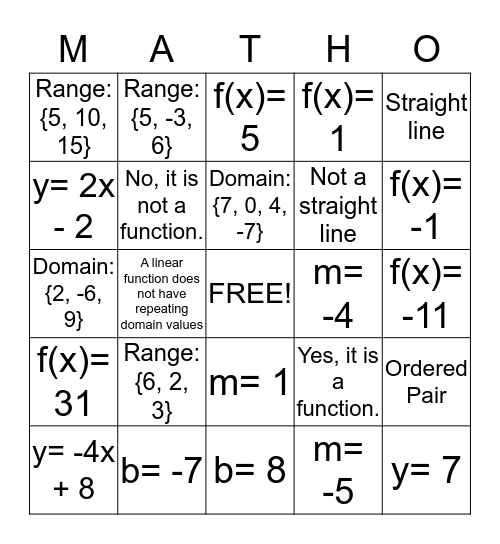# Domain, Range, Functions, & SlopeThis bingo card has a free space and 24 words: Range: {6, 2, 3}, b= -7, Domain: {7, 0, 4, -7}, y= -4x + 8, Straight line, Range: {5, 10, 15}, y= 2x - 2, Range: {5, -3, 6}, m= -4, m= 1, Not a straight line, Domain: {2, -6, 9}, Yes, it is a function., Ordered Pair, No, it is not a function., m= -5, f(x)= -11, f(x)= 5, f(x)= -1, A linear function does not have repeating domain values, y= 7, f(x)= 31, f(x)= 1 and b= 8.

## Play Online